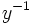# Commutator

## Definition

### Definition with symbols

The term commutator is used in group theory in two senses: the commutator map (typically with respect to the left action convention), and the set of possible images of that map. The commutator map is the following map:$\! (x,y) \mapsto xyx^{-1}y^{-1}$

And is denoted by outside square brackets (that is, the commutator of$x$ and$y$ is denoted as$[x,y]$). The image of this map is termed the commutator of$x$ and$y$.

An element of the group is termed a commutator if it occurs as the commutator of some two elements of the group.

Sometimes the commutator map is defined somewhat differently (typically with respect to the right action convention), i.e., as:$\! (x,y) \mapsto x^{-1}y^{-1}xy$

Although this alters the commutator map, it does not alter the set of elements that are commutators, because the commutator of$x$ and$y$ in one definition becomes the commutator of$x^{-1}$ and$y^{-1}$ in the other definition.

## Facts

### Identity element is a commutator

In fact, whenever$xy = yx$,$[x,y] = e$. Hence, in particular, the commutator of any element with itself is the identity element.

### Inverse of a commutator is a commutator

The inverse of the commutator$[x,y]$ is the commutator$[y,x]$ (this statement is true regardless of which definition of commutator we follow).

### Product of commutators need not be a commutator

In general, a product of commutators need not be a commutator.

## Iterated commutators

Since the commutator of two elements of the group is itself an element of the group, it can be treated as one of the inputs to the commutator map yet again. This allows us to construct iterated commutators.

A basic commutator is a word that can be expressed purely by iterating the commutator operations.

A simple commutator is an iterated commutator that can be expressed as a left-normed commutator, i.e., a commutator of the form:$[[[\dots [ x_1, x_2 ], x_3 ] \dots, x_n]$

## Subgroups related to the commutator

The subgroup generated by all the commutators is termed the derived subgroup.